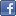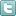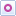EE Times-India > Memory/Storage

Memory/Storage# Grasp floating-point data in embedded software

Posted: 05 Oct 2015Print Version

Keywords:floating point  integer arithmetic  CPUs  coding  C

However, this approach, whilst superficially simple and straightforward, is rather inflexible and limits the range of values that may be represented. Mathematicians and scientists use a format for floating point numbers that is commonly called "Scientific Format", where the value is represented by a value greater than or equal to 1.0 but less than 10.0 (the mantissa) is multiplied by a power of 10 (the exponent). So, 1234 would be shown as 1.234 x 103 – commonly written 1.234e3 The same approach is normally used for computer representation of floating point, except that the mantissa is normally less than 1.0 and the exponent is a power of 2.

Historically, when all floating point operations were performed by software, a wide variety of format variations were in use, which were defined by computer manufacturers and compiler developers. Hardware floating point followed the same path initially, with each manufacturer offering their own variant. Nowadays, however, floating point format is standardised and IEEE 754-1985 is used almost universally. Again, it is useful to understand the principles of floating point formats, even if this knowledge is not exploited every day.

The standard describes both a single-precision (32bit) and a double-precision (64bit) variants. The discussion here will be confined to single-precision; double-precision uses exactly the same ideas.

A floating point value is represented by three fields: sign (1bit), exponent (8bit) and the mantissa (23bit).To formulate a number, the fields are employed as follows:
• The mantissa field is set to a value so that there is always a 1 before the binary point, so this is omitted, thus gaining an extra bit of precision. (The value 0 is an exception.)
• The exponent is set to a value which is the necessary exponent plus a bias of 127.
• The sign is set to 0 for positive numbers or 1 for negative.

For example, 14.2510 is 1110.012. This can be rewritten 1.110012 x 23. So, the floating point fields are assigned:
• The mantissa is .11001 after removing the leading 1.
• The exponent is 3 + the bias of 127, which is 130 (100000102).
• The sign is 0 as the number is positive

The resulting floating point representation looks like this:

0 | 1000 0010 | 110 0100 0000 0000 0000 0000

If this 32bit value were displayed in hex, it would be 0x41640000.

Conclusions
Broadly speaking, floating point should only be used if it is essential and only after every creative way to do the calculations using integers has been investigated and eliminated.

Colin Walls has over thirty years experience in the electronics industry, largely dedicated to embedded software. A frequent presenter at conferences and seminars and author of numerous technical articles and two books on embedded software, Colin is an embedded software technologist with Mentor Embedded (the Mentor Graphics Embedded Software Division), and is based in the UK.

 Related Articles Editor's Choice
Comment on "Grasp floating-point data in embedde..."

Top Ranked Articles

WebinarsVisit Asia Webinars to learn about the latest in technology and get practical design tips.

Search EE Times India
Services

Go to topConnect on FacebookFollow us on TwitterFollow us on Orkut
﻿